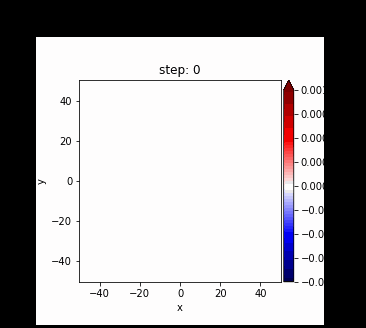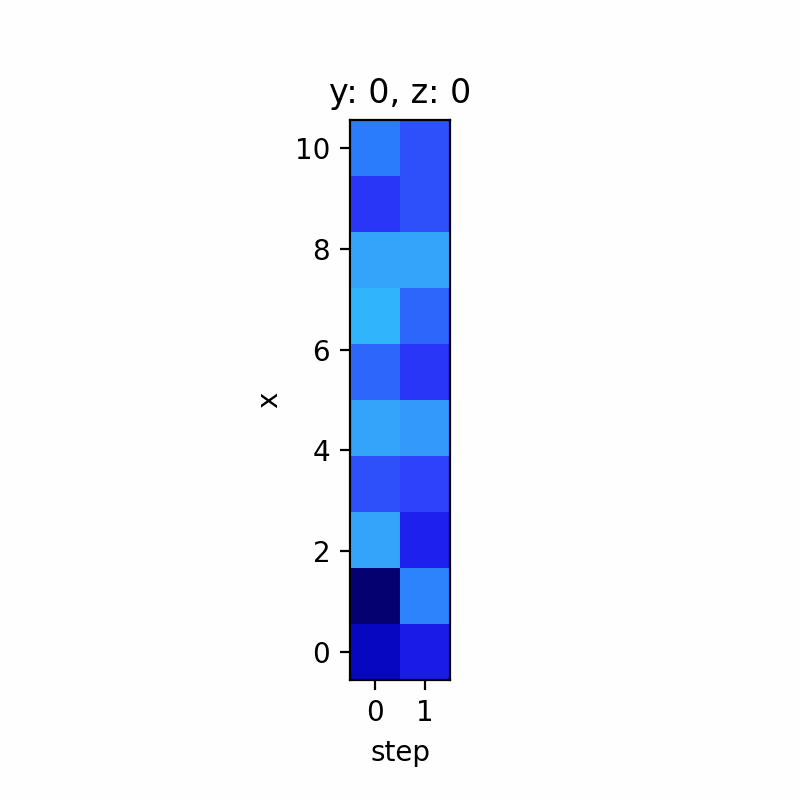# How to port the plot parameters from bokeh holoviews Image to matplotlib gif

Hello,
How can I port the plot parameters from bokeh holoviews Image to a matplotlib gif. I know pretty sure that it needs to be possible, because I did it yesterday (s. screenshot from gif:the plot params, such as the colorbar were created with bokeh)
, but somehow I can’t reproduce it anymore, although the code is the same.

The relevant code:

``````rand, x, y, z, step = np.random.randn(2, 10, 10, 10), np.linspace(0, 10, 10), np.linspace(0, 10, 10), np.linspace(0, 10, 10), [0, 1]
xa2 = xr.DataArray(rand, coords=dict(step=step, x=x, y=y, z=z, )
, dims=["step", "x", "y", "z"], name="field2")
hv_dst = hv.Dataset(xa2)
hv_imt = hv_dst.to(hv.Image)
fig = hv.render(hv_imt.opts(colorbar=True, width=500, height=400, cmap='seismic', clim=(-10**-3, 10**-3)), backend='matplotlib')
test2 = fig.save("testissue.gif", fps=0.5, dpi=200,)
``````

I would have expected that testissue.gif contained a `colorbar` and so on, but it doesn’t…

I also tried with `dynamic=True`, but didn’t help.No `colorbar` can be seen, the `colormap` is not `seismic` and so on…
I was able to fix it with `hv.extension('matplotlib')` instead of `hv.extension('bokeh' ,'matplotlib')`.
And then `hv.save(hv_imt.opts(colorbar=True, fig_size=500, cmap='seismic', clim=(-10**-3, 10**-3)), fmt="gif", backend="matplotlib", filename="test3", fps=1)`, without needing to `render`.